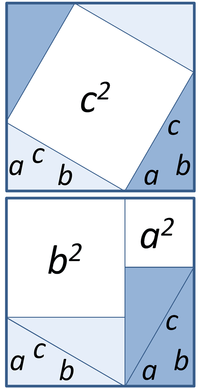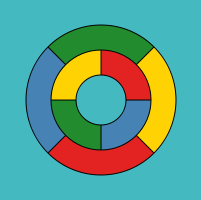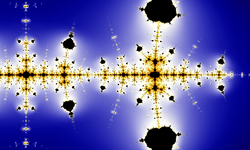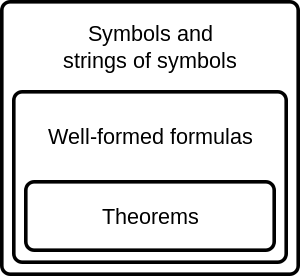Theorem

For the Italian film, see Teorema (film).The Pythagorean theorem has at least 370 known proofs

In mathematics, a theorem is a statement that has been proved on the basis of previously established statements, such as other theorems—and generally accepted statements, such as axioms. A theorem is a logical consequence of the axioms. The proof of a mathematical theorem is a logical argument for the theorem statement given in accord with the rules of a deductive system. The proof of a theorem is often interpreted as justification of the truth of the theorem statement. In light of the requirement that theorems be proved, the concept of a theorem is fundamentally deductive, in contrast to the notion of a scientific law, which is experimental.

Many mathematical theorems are conditional statements. In this case, the proof deduces the conclusion from conditions called hypotheses or premises. In light of the interpretation of proof as justification of truth, the conclusion is often viewed as a necessary consequence of the hypotheses, namely, that the conclusion is true in case the hypotheses are true, without any further assumptions. However, the conditional could be interpreted differently in certain deductive systems, depending on the meanings assigned to the derivation rules and the conditional symbol.

Although they can be written in a completely symbolic form, for example, within the propositional calculus, theorems are often expressed in a natural language such as English. The same is true of proofs, which are often expressed as logically organized and clearly worded informal arguments, intended to convince readers of the truth of the statement of the theorem beyond any doubt, and from which a formal symbolic proof can in principle be constructed. Such arguments are typically easier to check than purely symbolic ones—indeed, many mathematicians would express a preference for a proof that not only demonstrates the validity of a theorem, but also explains in some way why it is obviously true. In some cases, a picture alone may be sufficient to prove a theorem. Because theorems lie at the core of mathematics, they are also central to its aesthetics. Theorems are often described as being "trivial", or "difficult", or "deep", or even "beautiful". These subjective judgments vary not only from person to person, but also with time: for example, as a proof is simplified or better understood, a theorem that was once difficult may become trivial. On the other hand, a deep theorem may be simply stated, but its proof may involve surprising and subtle connections between disparate areas of mathematics. Fermat's Last Theorem is a particularly well-known example of such a theorem.

Informal account of theorems

Logically, many theorems are of the form of an indicative conditional: if A, then B. Such a theorem does not assert B, only that B is a necessary consequence of A. In this case A is called the hypothesis of the theorem (note that "hypothesis" here is something very different from a conjecture) and B the conclusion (formally, A and B are termed the antecedent and consequent). The theorem "If n is an even natural number then n/2 is a natural number" is a typical example in which the hypothesis is "n is an even natural number" and the conclusion is "n/2 is also a natural number".

To be proved, a theorem must be expressible as a precise, formal statement. Nevertheless, theorems are usually expressed in natural language rather than in a completely symbolic form, with the intention that the reader can produce a formal statement from the informal one.

It is common in mathematics to choose a number of hypotheses within a given language and declare that the theory consists of all statements provable from these hypotheses. These hypotheses form the foundational basis of the theory and are called axioms or postulates. The field of mathematics known as proof theory studies formal languages, axioms and the structure of proofs.Some theorems are "trivial", in the sense that they follow from definitions, axioms, and other theorems in obvious ways and do not contain any surprising insights. Some, on the other hand, may be called "deep", because their proofs may be long and difficult, involve areas of mathematics superficially distinct from the statement of the theorem itself, or show surprising connections between disparate areas of mathematics. A theorem might be simple to state and yet be deep. An excellent example is Fermat's Last Theorem, and there are many other examples of simple yet deep theorems in number theory and combinatorics, among other areas.

Other theorems have a known proof that cannot easily be written down. The most prominent examples are the four color theorem and the Kepler conjecture. Both of these theorems are only known to be true by reducing them to a computational search that is then verified by a computer program. Initially, many mathematicians did not accept this form of proof, but it has become more widely accepted. The mathematician Doron Zeilberger has even gone so far as to claim that these are possibly the only nontrivial results that mathematicians have ever proved. Many mathematical theorems can be reduced to more straightforward computation, including polynomial identities, trigonometric identities and hypergeometric identities.

Provability and theoremhood

To establish a mathematical statement as a theorem, a proof is required, that is, a line of reasoning from axioms in the system (and other, already established theorems) to the given statement must be demonstrated. However, the proof is usually considered as separate from the theorem statement. Although more than one proof may be known for a single theorem, only one proof is required to establish the status of a statement as a theorem. The Pythagorean theorem and the law of quadratic reciprocity are contenders for the title of theorem with the greatest number of distinct proofs.

Relation with scientific theories

Theorems in mathematics and theories in science are fundamentally different in their epistemology. A scientific theory cannot be proved; its key attribute is that it is falsifiable, that is, it makes predictions about the natural world that are testable by experiments. Any disagreement between prediction and experiment demonstrates the incorrectness of the scientific theory, or at least limits its accuracy or domain of validity. Mathematical theorems, on the other hand, are purely abstract formal statements: the proof of a theorem cannot involve experiments or other empirical evidence in the same way such evidence is used to support scientific theories.The Collatz conjecture: one way to illustrate its complexity is to extend the iteration from the natural numbers to the complex numbers. The result is a fractal, which (in accordance with universality) resembles the Mandelbrot set.

Nonetheless, there is some degree of empiricism and data collection involved in the discovery of mathematical theorems. By establishing a pattern, sometimes with the use of a powerful computer, mathematicians may have an idea of what to prove, and in some cases even a plan for how to set about doing the proof. For example, the Collatz conjecture has been verified for start values up to about 2.88 × 1018. The Riemann hypothesis has been verified for the first 10 trillion zeroes of the zeta function. Neither of these statements is considered proved.

Such evidence does not constitute proof. For example, the Mertens conjecture is a statement about natural numbers that is now known to be false, but no explicit counterexample (i.e., a natural number n for which the Mertens function M(n) equals or exceeds the square root of n) is known: all numbers less than 1014 have the Mertens property, and the smallest number that does not have this property is only known to be less than the exponential of 1.59 × 1040, which is approximately 10 to the power 4.3 × 1039. Since the number of particles in the universe is generally considered less than 10 to the power 100 (a googol), there is no hope to find an explicit counterexample by exhaustive search.

Note that the word "theory" also exists in mathematics, to denote a body of mathematical axioms, definitions and theorems, as in, for example, group theory. There are also "theorems" in science, particularly physics, and in engineering, but they often have statements and proofs in which physical assumptions and intuition play an important role; the physical axioms on which such "theorems" are based are themselves falsifiable.

Terminology

A number of different terms for mathematical statements exist, these terms indicate the role statements play in a particular subject. The distinction between different terms is sometimes rather arbitrary and the usage of some terms has evolved over time.

• An axiom or postulate is a statement that is accepted without proof and regarded as fundamental to a subject. Historically these have been regarded as "self-evident", but more recently they are considered assumptions that characterize the subject of study. In classical geometry, axioms are general statements, while postulates are statements about geometrical objects. A definition is also accepted without proof since it simply gives the meaning of a word or phrase in terms of known concepts.
• An unproved statement that is believed true is called a conjecture (or sometimes a hypothesis, but with a different meaning from the one discussed above). To be considered a conjecture, a statement must usually be proposed publicly, at which point the name of the proponent may be attached to the conjecture, as with Goldbach's conjecture. Other famous conjectures include the Collatz conjecture and the Riemann hypothesis. On the other hand, Fermat's last theorem has always been known by that name, even before it was proved; it was never known as "Fermat's conjecture".
• A proposition is a theorem of no particular importance. This term sometimes connotes a statement with a simple proof, while the term theorem is usually reserved for the most important results or those with long or difficult proofs. In classical geometry, a proposition may be a construction that satisfies given requirements; for example, Proposition 1 in Book I of Euclid's elements is the construction of an equilateral triangle.
• A lemma is a "helping theorem", a proposition with little applicability except that it forms part of the proof of a larger theorem. In some cases, as the relative importance of different theorems becomes more clear, what was once considered a lemma is now considered a theorem, though the word "lemma" remains in the name. Examples include Gauss's lemma, Zorn's lemma, and the Fundamental lemma.
• A corollary is a proposition that follows with little proof from another theorem or definition. Also a corollary is used for a theorem restated for a more restricted special case. For example, the theorem that all angles in a rectangle are right angles has as corollary that all angles in a square (a special case of a rectangle) are right angles.
• A converse of a theorem is a statement formed by interchanging what is given in a theorem and what is to be proved. For example, the isosceles triangle theorem states that if two sides of a triangle are equal then two angles are equal. In the converse, the given (that two sides are equal) and what is to be proved (that two angles are equal) are swapped, so the converse is the statement that if two angles of a triangle are equal then two sides are equal. In this example, the converse can be proved as another theorem, but this is often not the case. For example, the converse to the theorem that two right angles are equal angles is the statement that two equal angles must be right angles, and this is clearly not always the case.
• A generalization is a theorem which includes a previously proved theorem as a special case and hence as a corollary.

There are other terms, less commonly used, that are conventionally attached to proved statements, so that certain theorems are referred to by historical or customary names. For example:

A few well-known theorems have even more idiosyncratic names. The division algorithm (see Euclidean division) is a theorem expressing the outcome of division in the natural numbers and more general rings. The Bézout's identity is a theorem asserting that the greatest common divisor of two numbers may be written as a linear combination of these numbers. The Banach–Tarski paradox is a theorem in measure theory that is paradoxical in the sense that it contradicts common intuitions about volume in three-dimensional space.

Layout

A theorem and its proof are typically laid out as follows:

Theorem (name of person who proved it and year of discovery, proof or publication).
Statement of theorem (sometimes called the proposition).
Proof.
Description of proof.
End mark.

The end of the proof may be signalled by the letters Q.E.D. (quod erat demonstrandum) or by one of the tombstone marks "□" or "∎" meaning "End of Proof", introduced by Paul Halmos following their usage in magazine articles.

The exact style depends on the author or publication. Many publications provide instructions or macros for typesetting in the house style.

It is common for a theorem to be preceded by definitions describing the exact meaning of the terms used in the theorem. It is also common for a theorem to be preceded by a number of propositions or lemmas which are then used in the proof. However, lemmas are sometimes embedded in the proof of a theorem, either with nested proofs, or with their proofs presented after the proof of the theorem.

Corollaries to a theorem are either presented between the theorem and the proof, or directly after the proof. Sometimes, corollaries have proofs of their own that explain why they follow from the theorem.

Lore

It has been estimated that over a quarter of a million theorems are proved every year.

The well-known aphorism, "A mathematician is a device for turning coffee into theorems", is probably due to Alfréd Rényi, although it is often attributed to Rényi's colleague Paul Erdős (and Rényi may have been thinking of Erdős), who was famous for the many theorems he produced, the number of his collaborations, and his coffee drinking.

The classification of finite simple groups is regarded by some to be the longest proof of a theorem. It comprises tens of thousands of pages in 500 journal articles by some 100 authors. These papers are together believed to give a complete proof, and several ongoing projects hope to shorten and simplify this proof. Another theorem of this type is the Four color theorem whose computer generated proof is too long for a human to read. It is certainly the longest known proof of a theorem whose statement can be easily understood by a layman.

Theorems in logic

Logic, especially in the field of proof theory, considers theorems as statements (called formulas or well formed formulas) of a formal language. The statements of the language are strings of symbols and may be broadly divided into nonsense and well-formed formulas. A set of deduction rules, also called transformation rules or rules of inference, must be provided. These deduction rules tell exactly when a formula can be derived from a set of premises. The set of well-formed formulas may be broadly divided into theorems and non-theorems. However, according to Hofstadter, a formal system often simply defines all its well-formed formula as theorems.

Different sets of derivation rules give rise to different interpretations of what it means for an expression to be a theorem. Some derivation rules and formal languages are intended to capture mathematical reasoning; the most common examples use first-order logic. Other deductive systems describe term rewriting, such as the reduction rules for λ calculus.

The definition of theorems as elements of a formal language allows for results in proof theory that study the structure of formal proofs and the structure of provable formulas. The most famous result is Gödel's incompleteness theorem; by representing theorems about basic number theory as expressions in a formal language, and then representing this language within number theory itself, Gödel constructed examples of statements that are neither provable nor disprovable from axiomatizations of number theory.This diagram shows the syntactic entities that can be constructed from formal languages. The symbols and strings of symbols may be broadly divided into nonsense and well-formed formulas. A formal language can be thought of as identical to the set of its well-formed formulas. The set of well-formed formulas may be broadly divided into theorems and non-theorems.

A theorem may be expressed in a formal language (or "formalized"). A formal theorem is the purely formal analogue of a theorem. In general, a formal theorem is a type of well-formed formula that satisfies certain logical and syntactic conditions. The notationis often used to indicate thatis a theorem.

Formal theorems consist of formulas of a formal language and the transformation rules of a formal system. Specifically, a formal theorem is always the last formula of a derivation in some formal system each formula of which is a logical consequence of the formulas that came before it in the derivation. The initially accepted formulas in the derivation are called its axioms, and are the basis on which the theorem is derived. A set of theorems is called a theory.

What makes formal theorems useful and of interest is that they can be interpreted as true propositions and their derivations may be interpreted as a proof of the truth of the resulting expression. A set of formal theorems may be referred to as a formal theory. A theorem whose interpretation is a true statement about a formal system is called a metatheorem.

Syntax and semantics

The concept of a formal theorem is fundamentally syntactic, in contrast to the notion of a true proposition, which introduces semantics. Different deductive systems can yield other interpretations, depending on the presumptions of the derivation rules (i.e. belief, justification or other modalities). The soundness of a formal system depends on whether or not all of its theorems are also validities. A validity is a formula that is true under any possible interpretation, e.g. in classical propositional logic validities are tautologies. A formal system is considered semantically complete when all of its tautologies are also theorems.

Derivation of a theorem

Main article: Formal proof

The notion of a theorem is very closely connected to its formal proof (also called a "derivation"). To illustrate how derivations are done, we will work in a very simplified formal system. Let us call oursIts alphabet consists only of two symbols { A, B } and its formation rule for formulas is:

Any string of symbols ofthat is at least three symbols long, and is not infinitely long, is a formula. Nothing else is a formula.

The single axiom ofis:

ABBA.

The only rule of inference (transformation rule) foris:

Any occurrence of "A" in a theorem may be replaced by an occurrence of the string "AB" and the result is a theorem.

Theorems inare defined as those formulae that have a derivation ending with that formula. For example,

1. ABBA (Given as axiom)
2. ABBBA (by applying the transformation rule)
3. ABBBAB (by applying the transformation rule)

is a derivation. Therefore, "ABBBAB" is a theorem ofThe notion of truth (or falsity) cannot be applied to the formula "ABBBAB" until an interpretation is given to its symbols. Thus in this example, the formula does not yet represent a proposition, but is merely an empty abstraction.

Two metatheorems ofare:

Every theorem begins with "A".
Every theorem has exactly two "A"s.

Theorems and theories

• Inference
• List of theorems
• Toy theorem
• Metamath – a language for developing strictly formalized mathematical definitions and proofs accompanied by a proof checker for this language and a growing database of thousands of proved theorems

Notes

1. Elisha Scott Loomis. "The Pythagorean proposition: its demonstrations analyzed and classified, and bibliography of sources for data of the four kinds of proofs" (PDF). Education Resources Information Center. Institute of Education Sciences (IES) of the U.S. Department of Education. Retrieved 2010-09-26. Originally published in 1940 and reprinted in 1968 by National Council of Teachers of Mathematics.
2. However, both theorems and scientific law are the result of investigations. See Heath 1897 Introduction, The terminology of Archimedes, p. clxxxii:"theorem (θεὼρνμα) from θεωρεἳν to investigate"
3. Petkovsek et al. 1996.
4. Wentworth, G.; Smith, D.E. (1913). "Art. 46, 47". Plane Geometry. Ginn & Co.
5. Wentworth & Smith Art. 50
6. Wentworth & Smith Art. 51
7. Follows Wentworth & Smith Art. 79
8. The word law can also refer to an axiom, a rule of inference, or, in probability theory, a probability distribution.
9. Hoffman 1998, p. 204.
10. Hoffman 1998, p. 7.
11. An enormous theorem: the classification of finite simple groups, Richard Elwes, Plus Magazine, Issue 41 December 2006.Look up theorem in Wiktionary, the free dictionary.